# Vectors and the Geometry of Space: The Cross Product(Edited)

In this video I go over further into Vectors and the Geometry of Space and this time cover the Cross Product. The cross product is a very useful concept and is defined such that it produces a vector that is perpendicular to 2 other vectors. This property is very useful in physics, especially when dealing with rotation and torque.

While the definition of the cross product involves a lot of algebra, I show that it can be made easier to both remember and calculate by writing it in determinant form. Furthermore, I show that the length of the cross product is equal to the parallelogram formed from 2 vectors. I also go over several other theorems and topics that follow from the cross product, such as the vector triple product and a number of properties of the cross product. This is a great in-depth introduction and examination of the cross product so make sure to watch this whole video!

The topics covered as well as their timestamps are listed below.

• Introduction: 0:00
• Calculus Book Reference: 0:44
• Sections in Calculus Book Chapter: 1:06
• Topics to Cover: 1:38
1. The Cross Product: 3:01
• Definition: 15:36
• Historical Note: Sir William Rowan Hamilton: 16:55
2. Determinants: 18:01
• Cross Product in Determinant Form: 24:48
• Example 1: 30:27
• Example 2: 33:38
3. Cross Product Orthogonality Proof: 36:50
• Theorem 1: 37:19
4. Right-Hand Rule: 43:07
5. Cross Product Length: 44:58
• Theorem 2: 45:23
6. Corollary: 1:11:47
7. Geometric Interpretation of Theorem 2: 1:15:19
• Example 3: 1:19:46
• Example 4: 1:31:44
8. Properties of the Cross Product: 1:35:03
• Theorem 3: 1:45:36
• Proof of Property 5: 1:47:33
9. Triple Products: 1:53:09
• Formula of a Parallelepiped: 2:01:08
• Example 5: 2:02:05
10. Torque: 2:07:00
• Example 6: 2:10:31
11. Exercises: 2:14:42
• Exercise 1: 2:15:20
• Exercise 2: 2:18:23
• Exercise 3: 2:22:12
• Exercise 4: 2:28:16
• Exercise 5: Vector Triple Product: 2:34:08

Watch video on:

View the full Vectors and Geometry of Space video series: https://www.youtube.com/playlist?list=PLai3U8-WIK0FjJpwnxwdrOR7L8Ul8VZoZ

# View Video Notes Below!

View these notes as an article: https://peakd.com/@mes
Subscribe via email: http://mes.fm/subscribe
Donate! :) https://mes.fm/donate
Subscribe to MES Truth: https://mes.fm/truth

Reuse of my videos:

• Feel free to make use of / re-upload / monetize my videos as long as you provide a link to the original video.

Fight back against censorship:

• Bookmark sites/channels/accounts and check periodically
• Remember to always archive website pages in case they get deleted/changed.

Recommended Books:

Join my forums!

Follow along my epic video series:

NOTE #1: If you don't have time to watch this whole video:

Browser extension recommendations:

# Vectors and the Geometry of Space: The Cross Product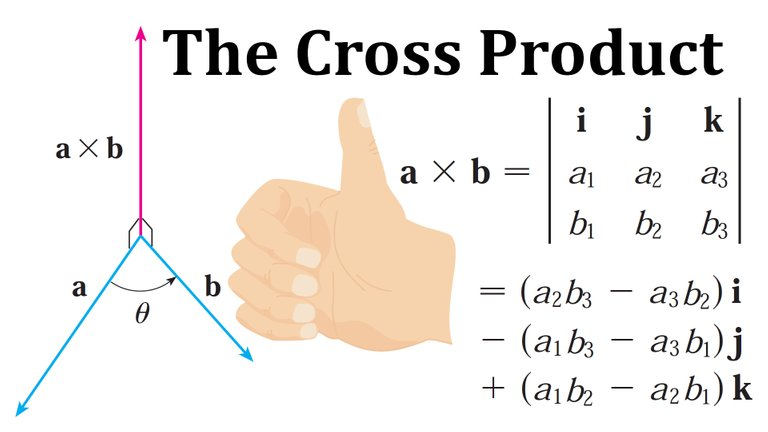## Calculus Book Reference

Note that I mainly follow along the following calculus book:

• Calculus: Early Transcendentals 7th Edition by James Stewart
• Note: In the earlier videos I used the 6th edition.

## Sections in Calculus Book Chapter

I have made a list of the sections in this particular chapter with links to the Hive post of the videos I have already finished.

Vectors and the Geometry of Space

1. 3-D Coordinate Systems
2. Vectors
3. The Dot Product
4. The Cross Product (Current Video)
• Discovery Project: The Geometry of a Tetrahedron
5. Equations of Lines and Planes
• Laboratory Project: Putting 3D in Perspective
7. Review
• True-False Quiz
8. Problems Plus

## Topics to Cover

Note that the timestamps will be included in the video description for each topic listed below.

1. The Cross Product
• Definition
• Historical Note: Sir William Rowan Hamilton
2. Determinants
• Cross Product in Determinant Form
• Example 1
• Example 2
3. Cross Product Orthogonality Proof
• Theorem 1
4. Right-Hand Rule
5. Cross Product Length
• Theorem 2
6. Corollary
7. Geometric Interpretation of Theorem 2
• Example 3
• Example 4
8. Properties of the Cross Product
• Theorem 3
• Proof of Property 5
9. Triple Products
• Formula of a Parallelepiped
• Example 5
10. Torque
• Example 6
11. Exercises
• Exercise 1
• Exercise 2
• Exercise 3
• Exercise 4
• Exercise 5: Vector Triple Product

# The Cross Product

Given two nonzero vectors a = <a1, a2, a3> and b = <b1, b2, b3>, it is very useful to be able to find a nonzero vector c that is perpendicular to both a and b, as we will see in the next section and in future chapters.

If c = <c1, c2, c3> is such a vector, then ac = 0 and bc = 0 (i.e. the dot product is 0 for perpendicular vectors) and so: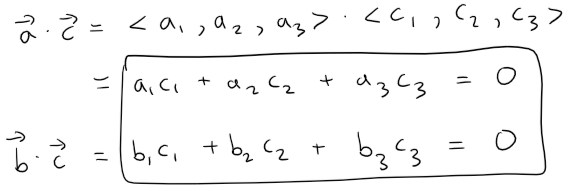To eliminate c3 we multiply the first equation by b3 and the second by a3 and subtract: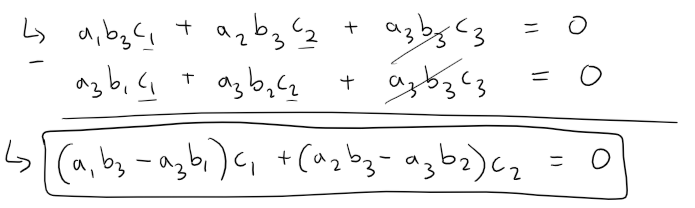The equation above has the form pc1 + qc2 = 0, for which an obvious solution is: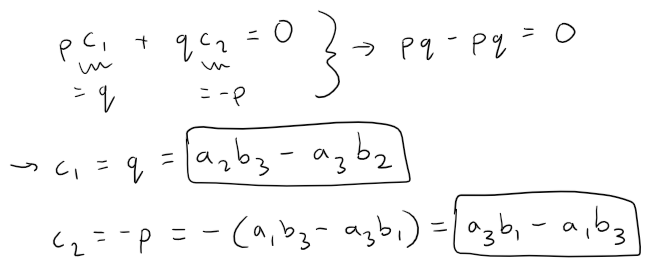Substituting these values into either of the original equations, we then get: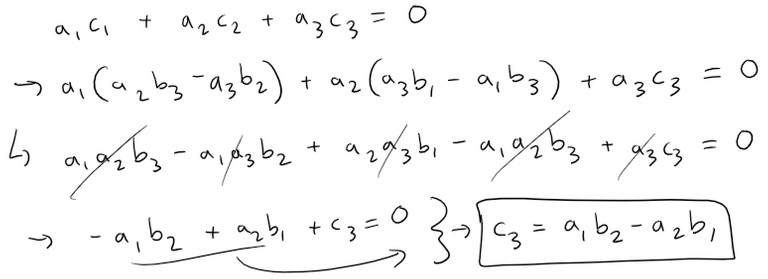This means that a vector perpendicular to both a and b is: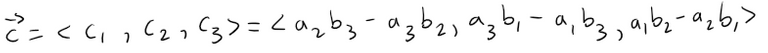The resulting vector is called the cross product of a and b and is denoted by a × b.

## Definition

If a = <a1, a2, a3> and b = <b1, b2, b3>, then the cross product of a and b is the vector: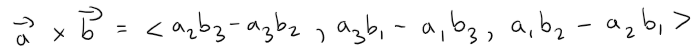Notice that the cross product a × b of two vectors a and b, unlike the dot product, is a vector.

For this reason it is also called the vector product.

Note that a × b is defined only when a and b are three-dimensional vectors.

## Historical Note: Sir William Rowan Hamilton

The cross product was invented by the Irish mathematician Sir William Rowan Hamilton (3/4 August 1805 to 2 September 1865), who had created a precursor of vectors, called quaternions.

When he was five years old Hamilton could read Latin, Greek, and Hebrew.

At the age eight he added French and Italian and when he was ten he could read Arabic and Sanskrit.

At the age of 21, while still an undergraduate at Trinity College in Dublin, Ireland, Hamilton was appointed Professor of Astronomy at the university and Royal Astronomer of Ireland!

# Determinants

In order to make the Definition easier to remember, we use the notation of determinants.

A determinant of order 2 is defined by: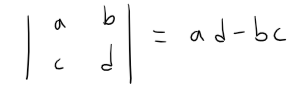For example: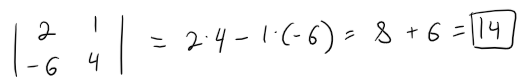A determinant of order 3 can be defined in terms of second-order determinants as follows:Observe that each term on the right side of the above equation involves a number ai in the first row of the determinant, and ai is multiplied by the second-order determinant obtained from the left side by deleting the row and column in which ai appears.

For example: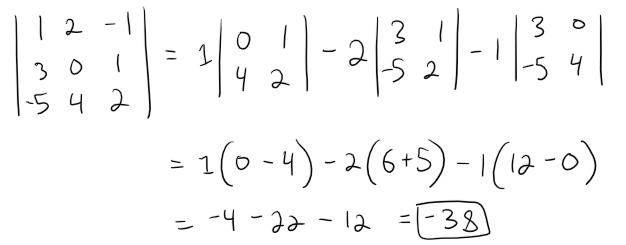## Cross Product in Determinant Form

If we now rewrite the Definition of the Cross Product using second-order determinants and the standard basis vectors i, j, and k, we see that the cross product of the vectors a = a1i + a2j + a3k and b = b1i + b2j + b3k is: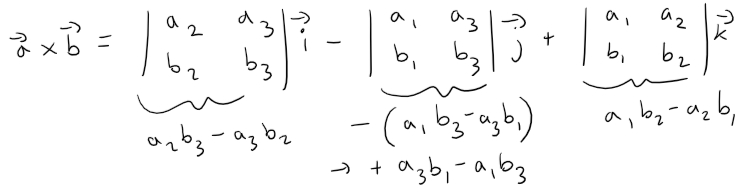In view of the similarity between the above equation and the 3rd-order determinant, we often write: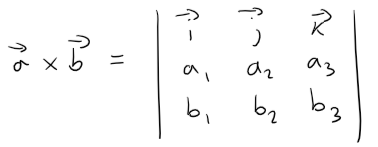Although the first row of the symbolic determinant in the above equation consists of vectors, if we expand it as if it were an ordinary determinant of order 3, we will obtain our standard basis / 2nd-order determinant form.

The symbolical determinant formula is probably the easiest way of remembering and computing cross products.

## Example 1

If a = <1, 3, 4> and b = < 2, 7, -5>, compute the cross product.

Solution: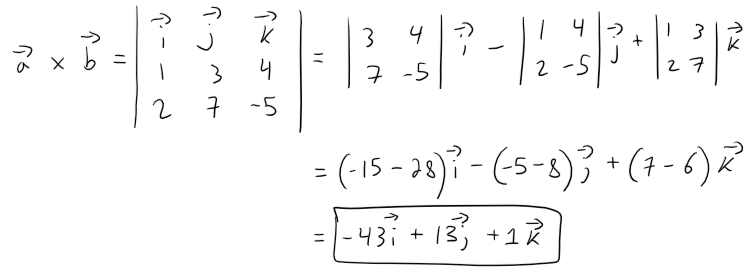## Example 2

Show that a × a = 0 for any vector a in V3.

Solution:

If a = <a1, a2, a3>, then: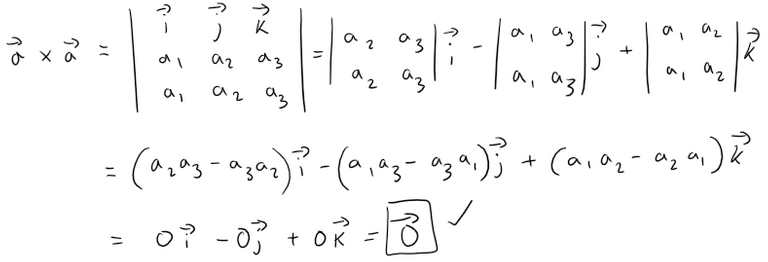# Cross Product Orthogonality Proof

We constructed the cross product a × b so that it would be perpendicular to both a and b.

This is one of the most important properties of a cross product, so let's emphasize and verify it in the following theorem and give a formal proof.

## Theorem 1

The vector a × b is orthogonal to both a and b.

Proof:

In order to show that a × b is orthogonal to a, we compute their dot product as follows: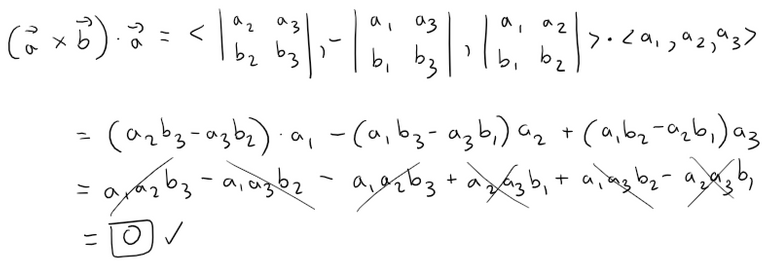A similar computation shows that (a × b) ⋅ b = 0.

Therefore a × b is orthogonal to both a and b.

# Right-Hand Rule

If a and b are represented by directed line segments with the same initial point (as in the figure below), then the above theorem says that the cross product a × b points in a direction perpendicular to the plane through a and b.

It turns out that the direction of a × b is given by the right-hand rule:

If the fingers of your right hand curl in the direction of a rotation (through an angle less than 180°) from a to b, then your thumb points in the direction of a × b.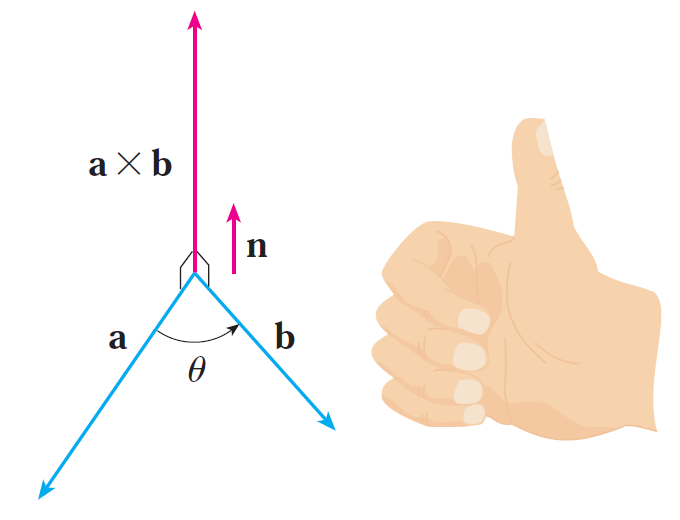# Cross Product Length

Now that we know the direction of the vector a × b, the remaining thing we need to complete its geometric description is its length |a × b|.

This is given by the following theorem.

## Theorem 2

If θ is the angle between a and b (so 0 ≤ θ ≤ π), then: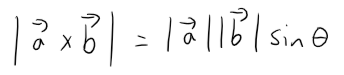Proof:

From the definitions of the cross product and length of a vector we have: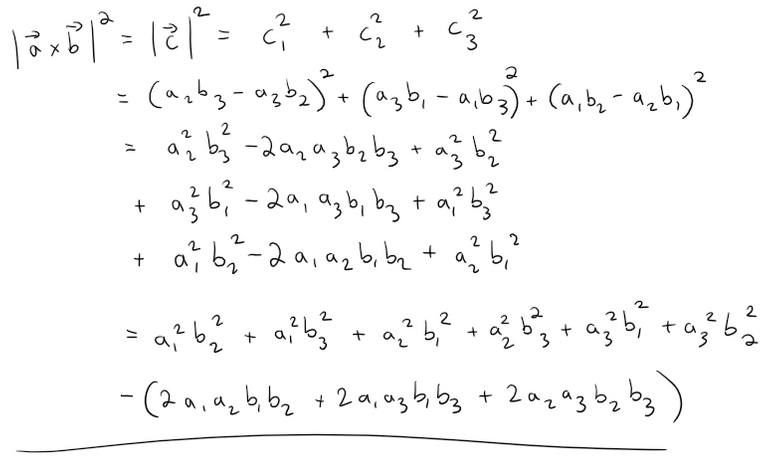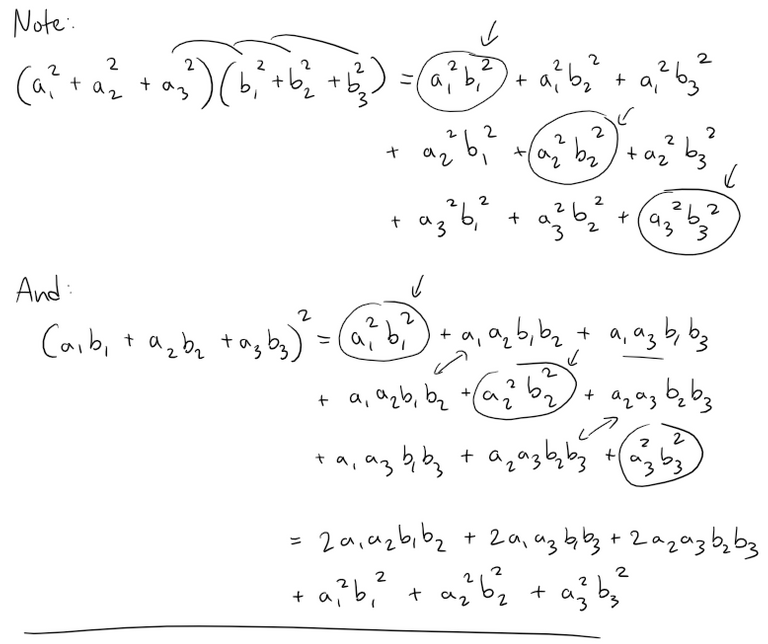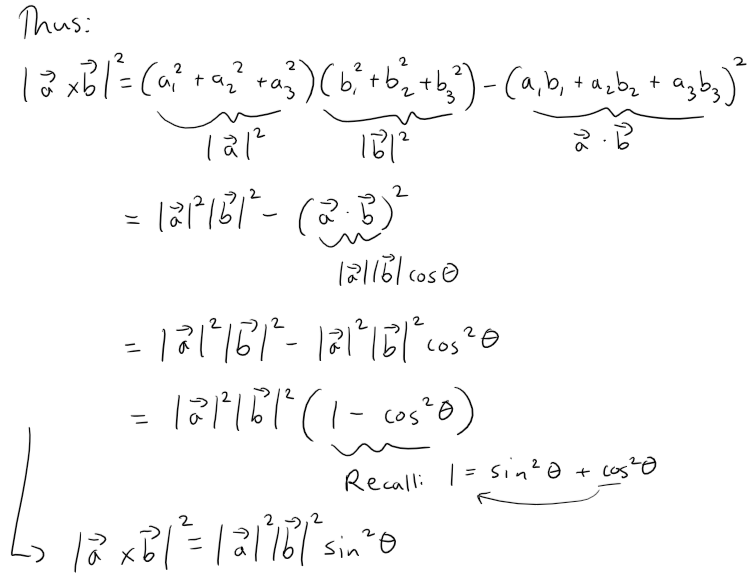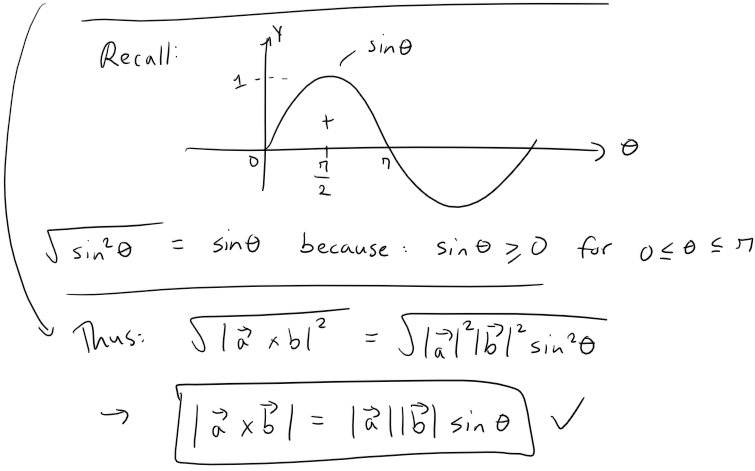Since a vector is completely determined by its magnitude and direction, we can now say that the cross product a × b is the vector that is perpendicular to both a and b, whose orientation is determined by the right-hand rule, and whose length is |a||b| sin θ.

In fact, that is exactly how physicists define a × b.

# Corollary

Two nonzero vectors a and b are parallel if and only if: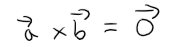Proof:

Two nonzero vectors a and b are parallel if and only if θ = 0 or π.

In either case sin θ = 0, so |a × b| = 0 and therefore a × b = 0.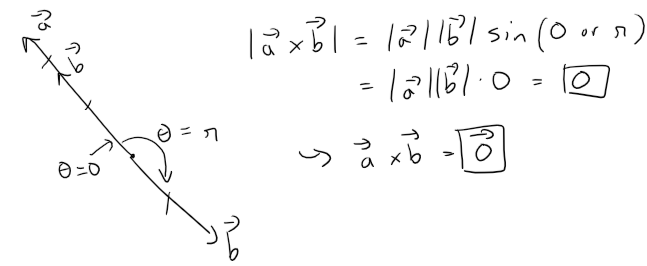# Geometric Characterization of Theorem 2

The geometric interpretation of Theorem 2 can be seen by looking at the figure below.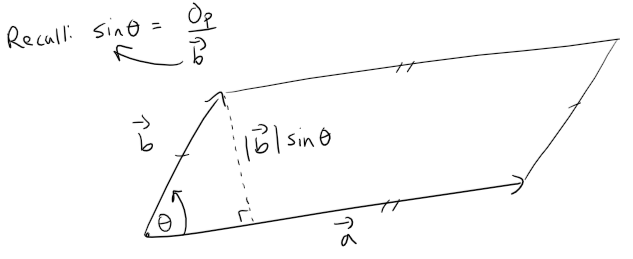If a and b are represented by directed line segments with the same initial point, then they determine a parallelogram with base |a|, altitude |b| sin θ, and area: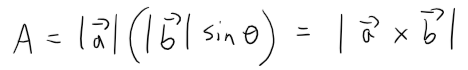Thus we have the following way of interpreting the magnitude of a cross product.

The length of the cross product a × b is equal to the area of the parallelogram determined by a and b.

## Example 3

Find a vector perpendicular to the plane that passes through the points P(1, 4, 6), Q(-2, 5, -1), and R(1, -1, 1).

Solution:

The vector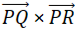is perpendicular to both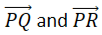and is therefore perpendicular to the plane through P, Q, and R.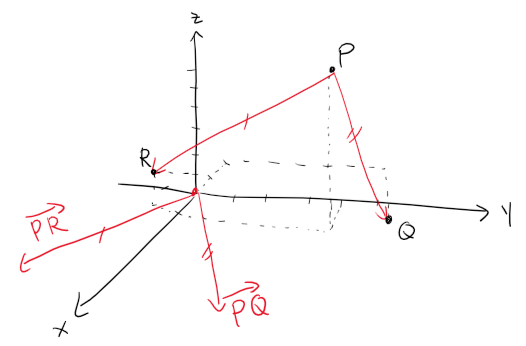Recall from my earlier video on Vectors that the position vector a of the point P can be written as follows: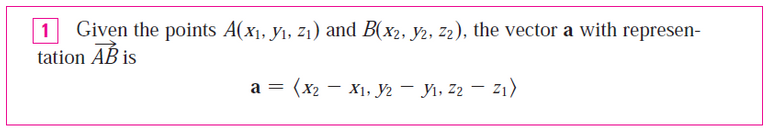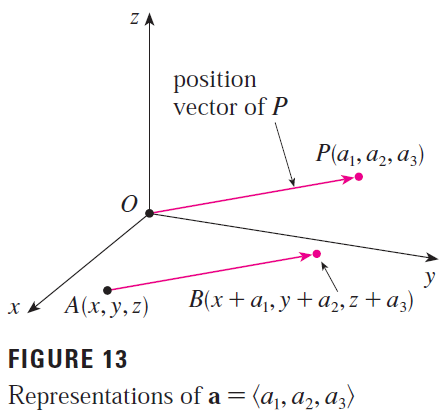Thus from the above formula, we know that the position vectors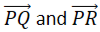are: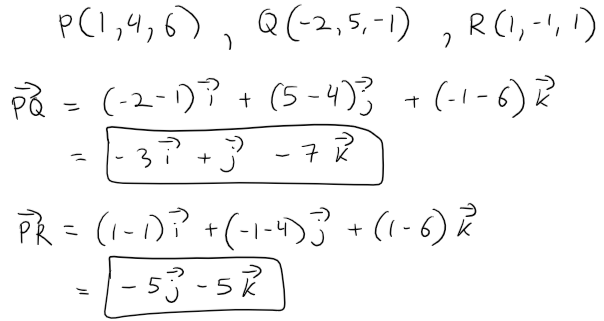We compute the cross product of these vectors: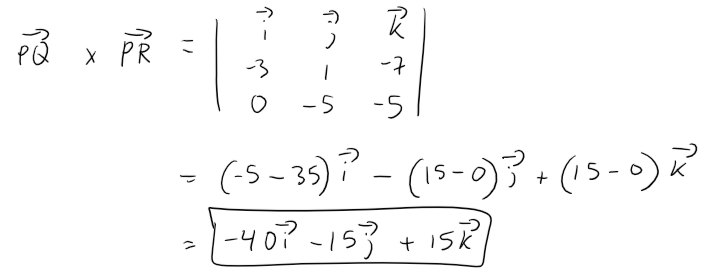So the vector <-40, -15, 15> is perpendicular to the given plane.

Any nonzero scalar multiple of this vector, such as <-8, -3, 3>, is also perpendicular to the plane.

Calculation Check:

-40/-8 = 5
-15/-3 = 5
15 / 3 = 5

## Example 4

Find the area of the triangle with vertices P(1, 4, 6), Q(-2, 5, -1), and R(1, -1, 1).

Solution:

In Example 3 we computed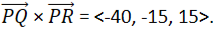The area of the parallelogram with adjacent sides PQ and PR is the length of this cross product.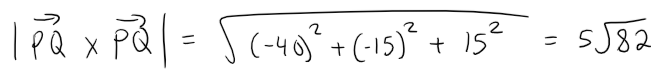Calculation Check:

(-40)^2 + (-15)^2 + 15^2 = 2,050
2,050 / 25 = 82

2,0501/2 = (25 * 82)1/2 = 5 * (82)1/2

The area of the triangle PQR is half the area of this parallelogram, that is, 5/2 (82)1/2.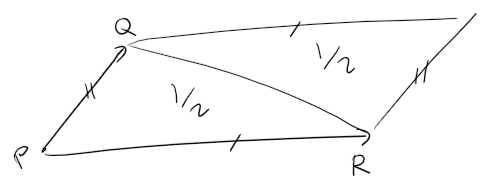# Properties of the Cross Product

If we apply Theorems 1 and 2 to the standard basis vectors i, j, and k using θ = π/2, (and the right-hand rule) we obtain: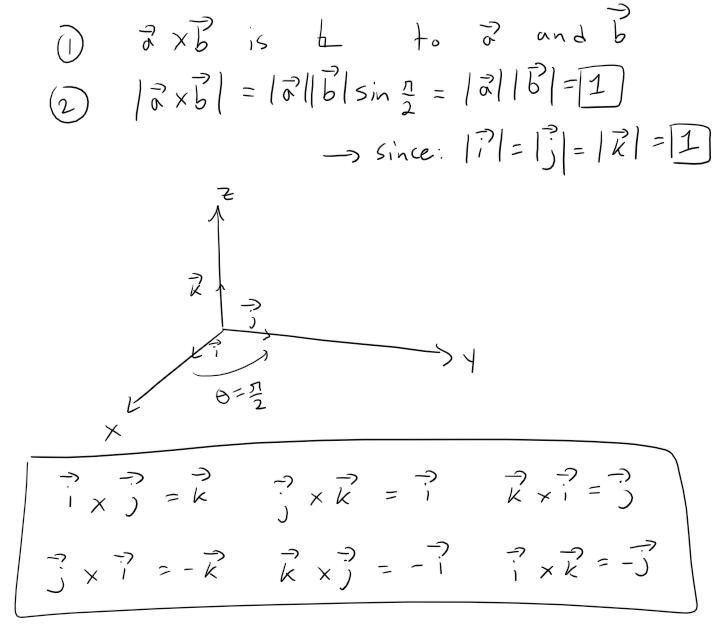Observe that: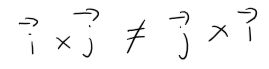Thus the cross product is not commutative.

Note also that: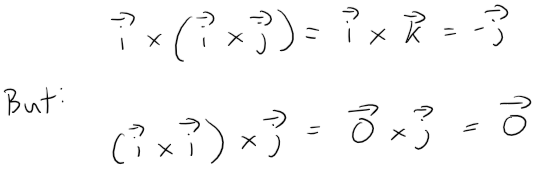So the associative law for multiplication does not usually hold; that is, in general: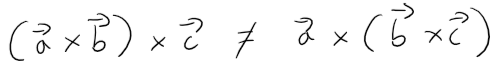However, some of the usual laws of algebra do hold for cross products.

The following theorem summarizes the properties of vector products.

## Theorem 3

If a, b, and c are vectors and c is a scalar, then:

1. a × b = -b × a
2. (ca) × b = c(a × b) = a × (cb)
3. a × (b + c) = a × b + a × c
4. (a + b) × c = a × c + b × c
5. a ⋅ (b × c) = (a × b) ⋅ c
6. a × (b × c) = (ac)b - (ab)c

These properties can be proved by writing the vectors in terms of their components and using the definition of a cross product.

We give the proof of Property 5 and leave the remaining proofs as exercises at the end of this video.

## Proof of Property 5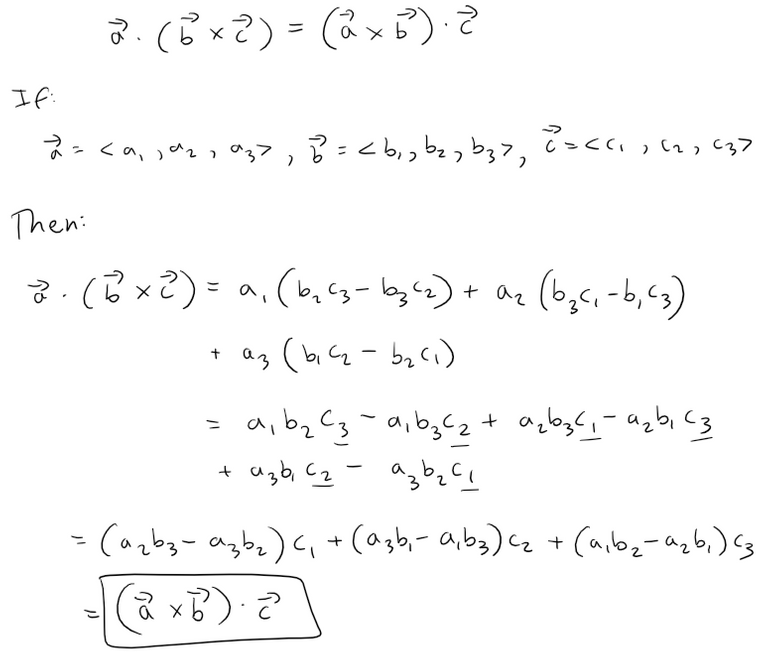# Triple Products

The product a ⋅ (b × c) that occurs in Property 5 is called the scalar triple product of the vectors a, b, and c.

Notice from the Property 5 Proof that we can write the scalar triple product as a determinant: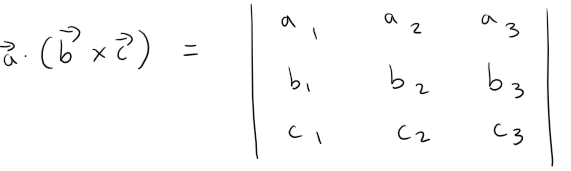The geometric significance of the scalar triple product can be seen by considering the parallelepiped determined by the vectors a, b, and c, as shown in the figure below.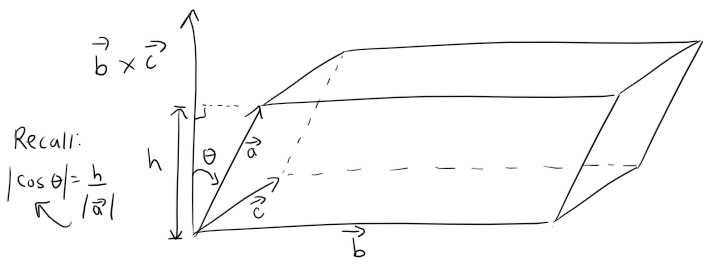The area of the base of the parallelogram is A = |b × c|.

If θ is the angle between a and b × c, then the height h = |a||cos θ|.

Note that we must use |cos θ| instead of cos θ in case θ > π/2.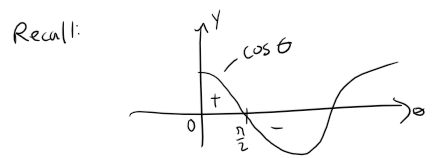Therefore the volume of the parallelepiped is: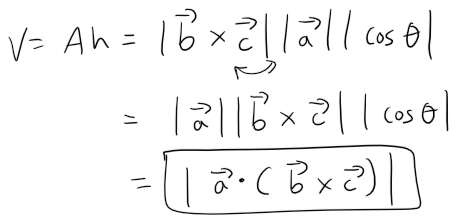Thus we have proved the following formula:

## Formula of a Parallelepiped

The volume of the parallelepiped determined by the vectors a, b, and c, is the magnitude of their scalar triple product: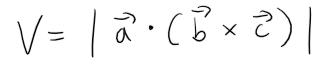If we use the above formula and discover that the volume of the parallelepiped determined by a, b, and c is 0, then the vectors must lie in the same plane; that is, they are coplanar.

## Example 5

Use the scalar triple product to show that the vectors a = <1, 4, -7>, b = <2, -1, 4>, and c = <0, -9, 18> are coplanar.

Solution:

We use the determinant formula to compute their scalar triple product.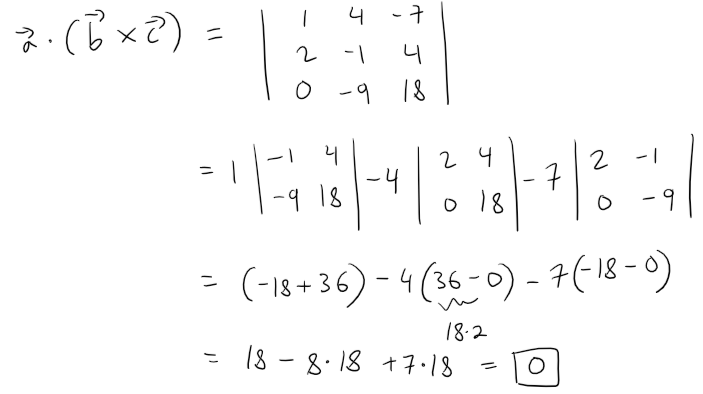Therefore, the volume of the parallelepiped determined by a, b, and c is 0.

This means that a, b, and c are coplanar.

The product a × (b × c) that occurs in Property 6 is called the vector triple product of a, b, and c.

Property 6 will be used to derive Kepler's First Law of planetary motion in my future video.

Its proof is shown in Exercise 5 at the end of this video.

# Torque

The idea of a cross product occurs often in physics.

In particular, we consider a force F acting on a rigid body at a point given by a position vector r.

For instance, if we tighten a bolt by applying a force to a wrench as in the figure below, we produce a turning effect.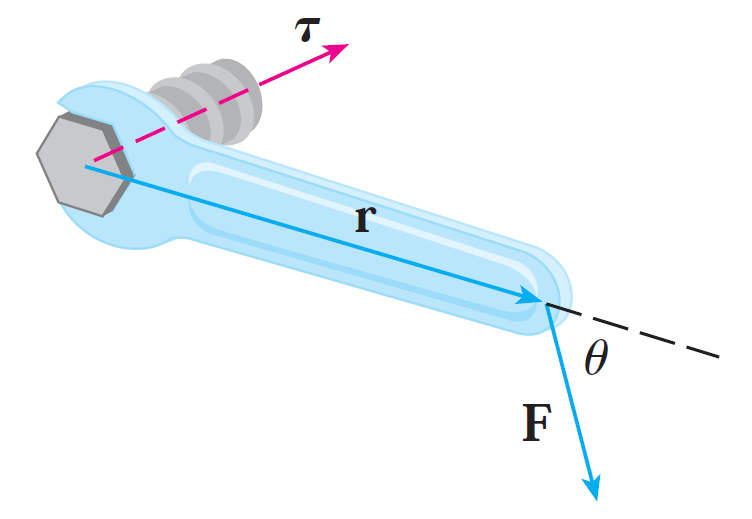The torque τ (relative to the origin) is defined to be the cross product of the position and force vectors: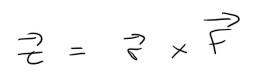and measures the tendency of the body to rotate about the origin.

The direction of the torque vector indicates the axis of rotation.

According to Theorem 2, the magnitude of the torque vector is: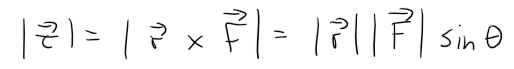where θ is the angle between the position and force vectors.

Observe that the only component of F that can cause a rotation is the one perpendicular to r, that is, |F| sin θ.

The magnitude of the torque is equal to the area of the parallelogram determined by r and F.

## Example 6

A bolt is tightened by applying a 40-N force to a 0.25-m wrench as shown in the figure below.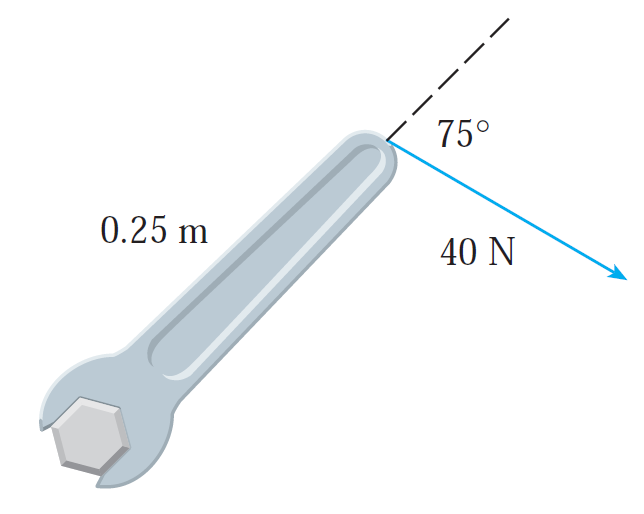Find the magnitude of the torque about the center of the bolt.

Solution:

The magnitude of the torque vector is: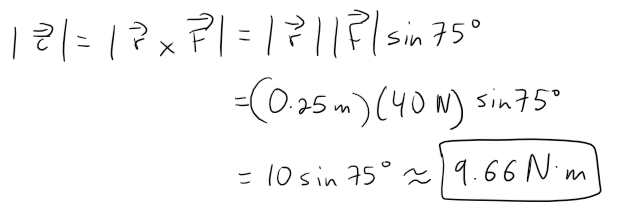Calculation check:

10*sin(75) = 9.659258262890683

If the bolt is right-threaded, then the torque vector itself is: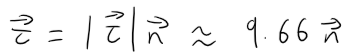where n is a unit vector directed down into the page, aka right-hand rule with the thumb pointing into the page and fingers curling in a clockwise rotation.

# Exercises

## Exercise 1

Prove Property 1 of Theorem 3:

a × b = -b × a

Solution: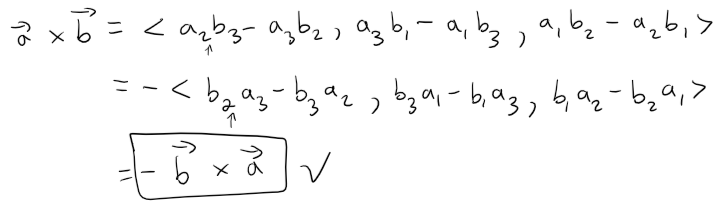## Exercise 2

Prove Property 2 of Theorem 3:

(ca) × b = c(a × b) = a × (cb)

Solution: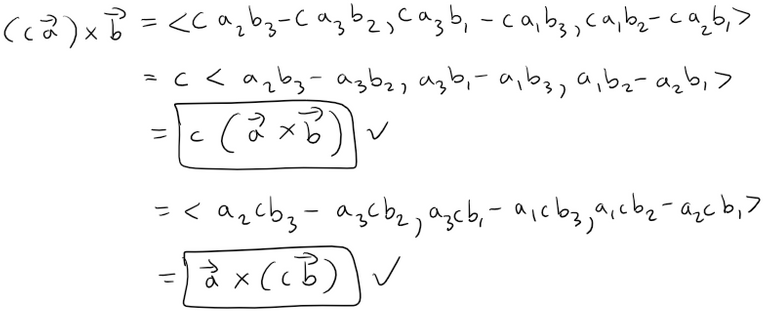## Exercise 3

Prove Property 3 of Theorem 3:

a × (b + c) = a × b + a × c

Solution: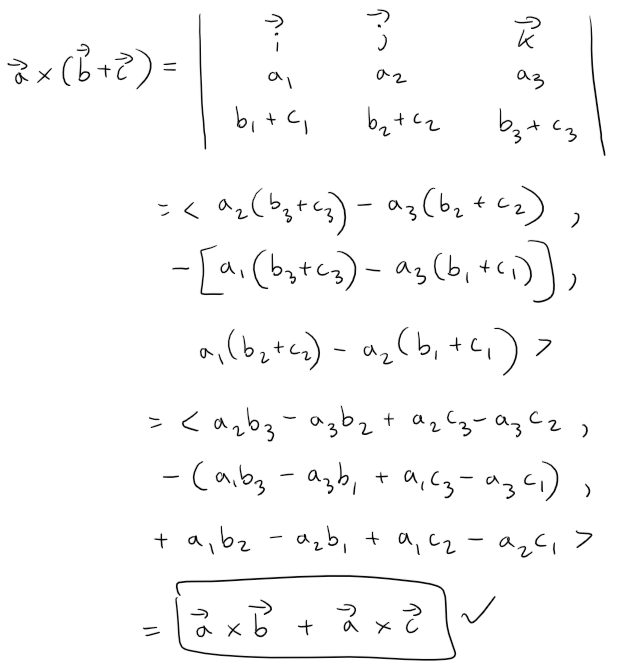## Exercise 4

Prove Property 4 of Theorem 3:

(a + b) × c = a × c + b × c

Solution: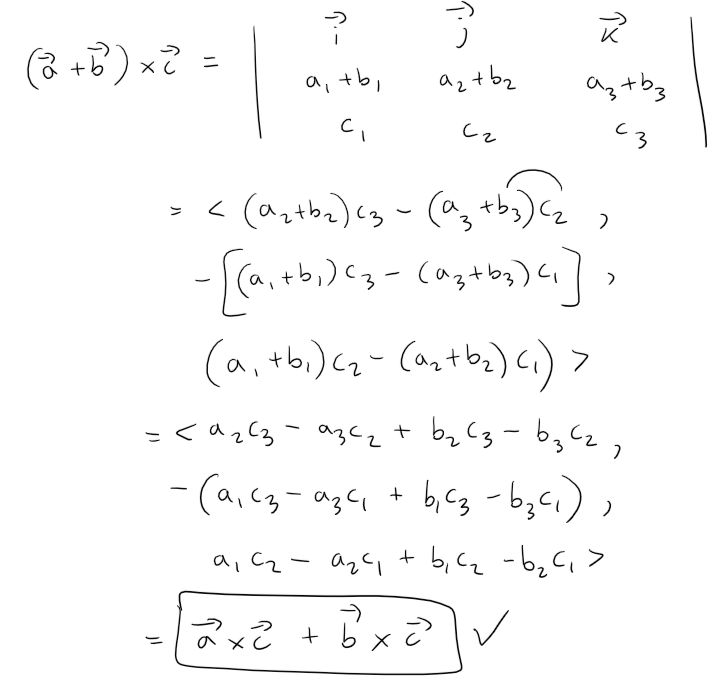## Exercise 5: Vector Triple Product

Prove Property 6 of Theorem 3:

a × (b × c) = (ac)b - (ab)c

Solution: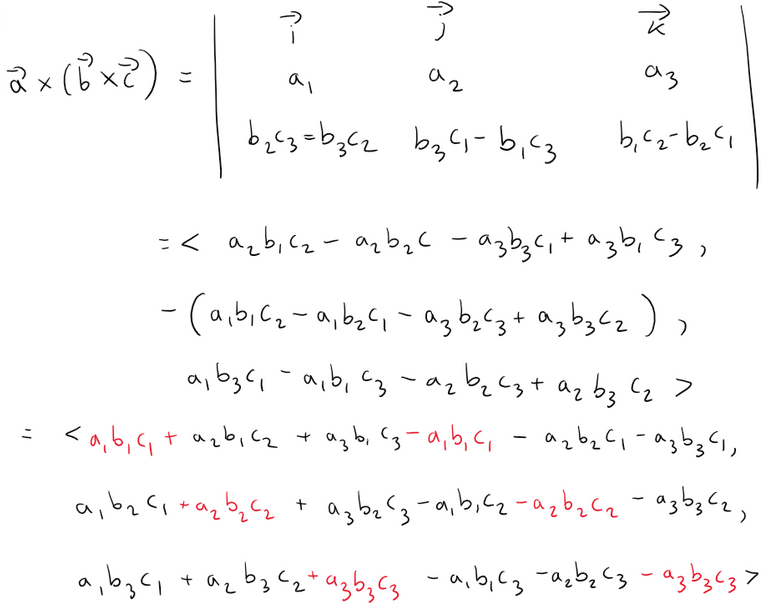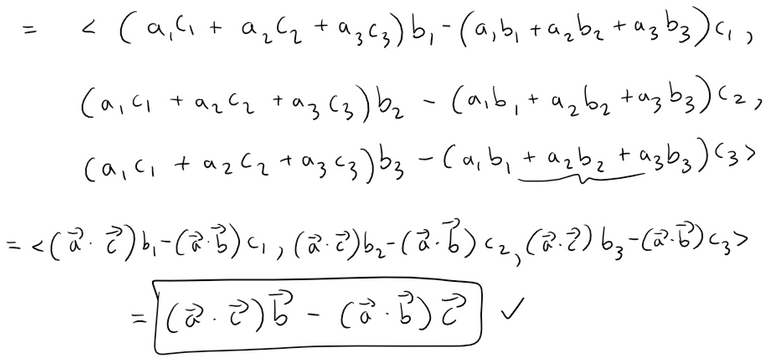0
0
0.000